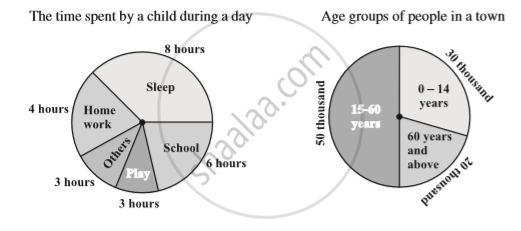Share

# Introduction of Circle Graph Or Pie Chart

Course

#### notes

The data represented in circular form as shown in below :These are called circle graphs. A circle graph shows the relationship between a whole and its parts. Here, the whole circle is divided into sectors. The size of each sector is proportional to the activity or information it represents.
For example, in the above graph, the proportion of the sector for hours spent in sleeping

= "number of sleeping hours" / "Whole day" = (8  "hours")/ (24  "hours") = 1/3
So, this sector is drawn as 1/3rd  part of the circle. A circle graph is also called a pie chart.

### Shaalaa.com

Pie Charts [00:04:29]
S
0%

S